Ex 4.5

Chapter 4 Class 12 Determinants
Serial order wise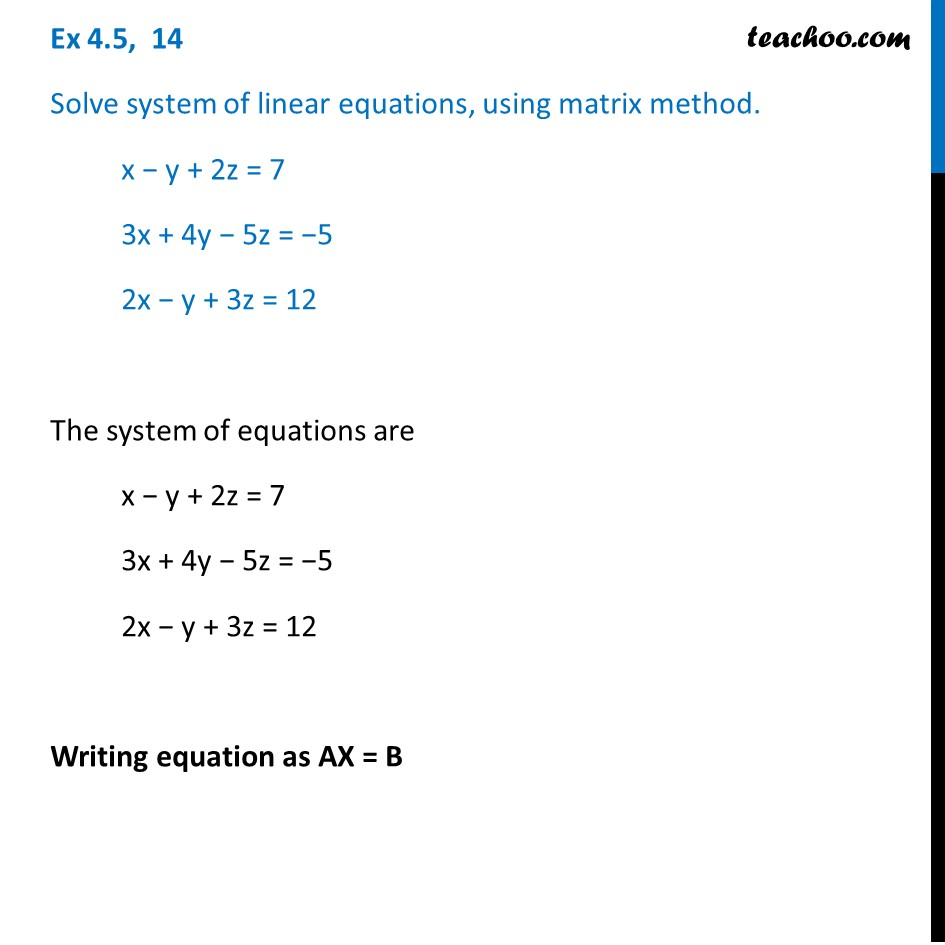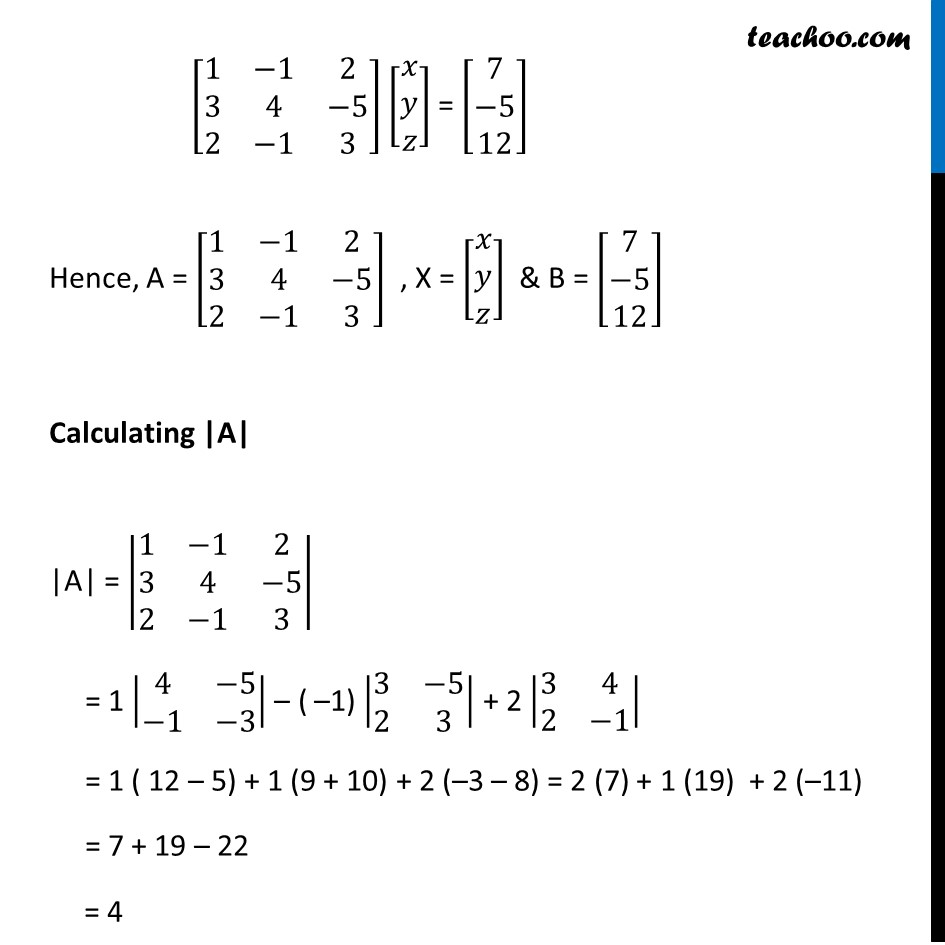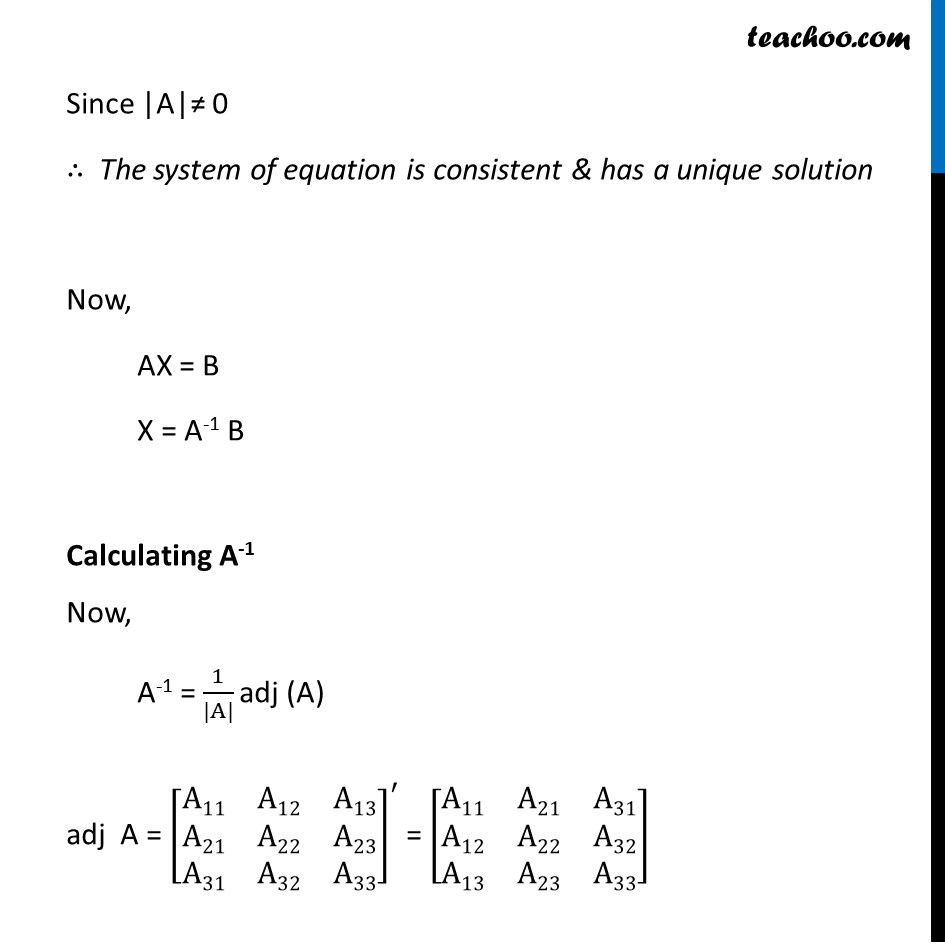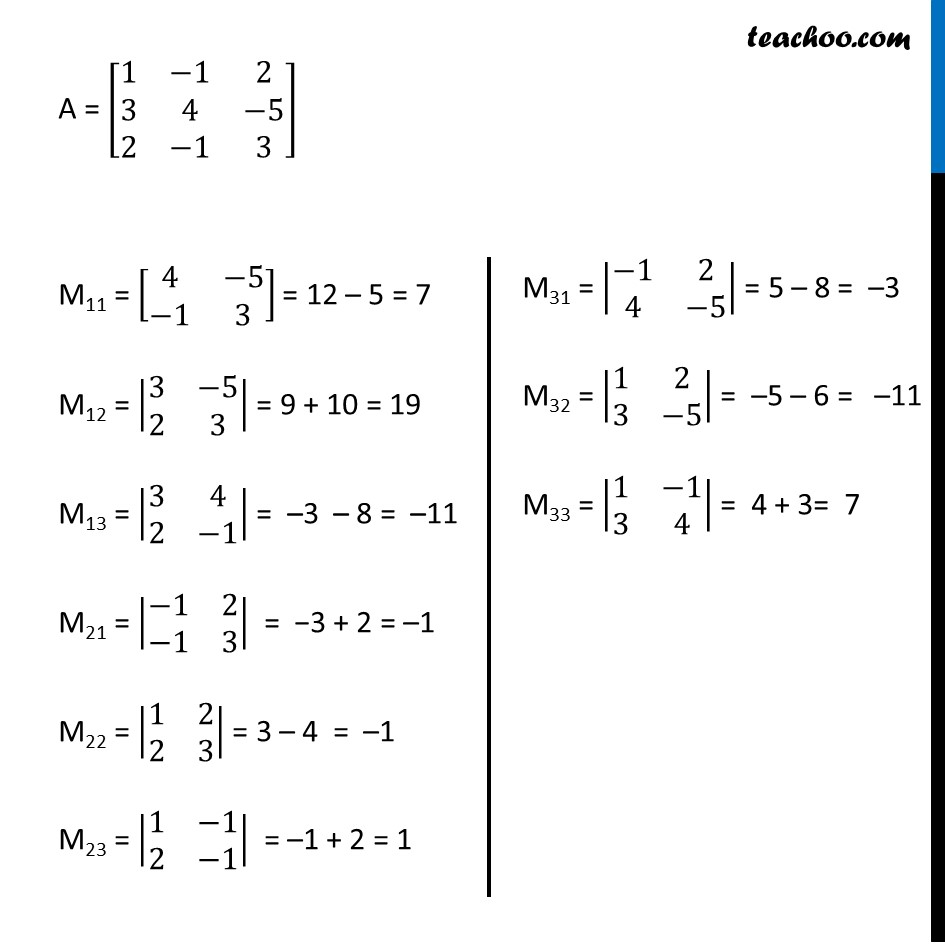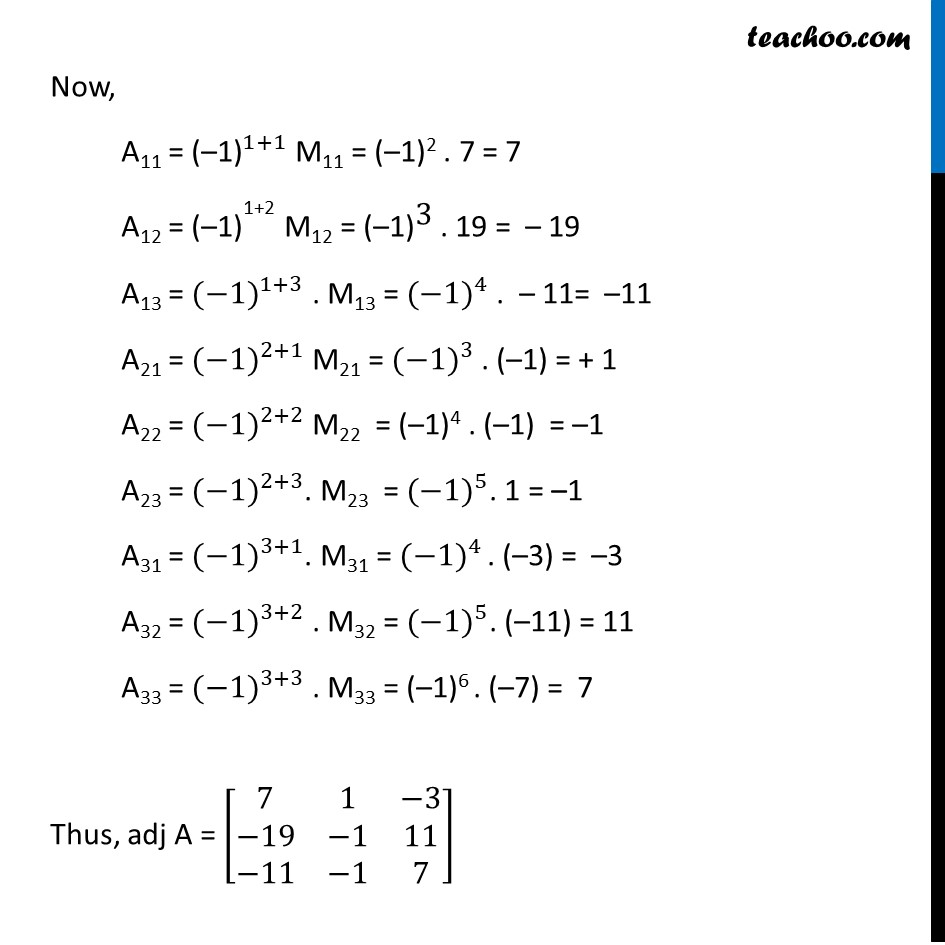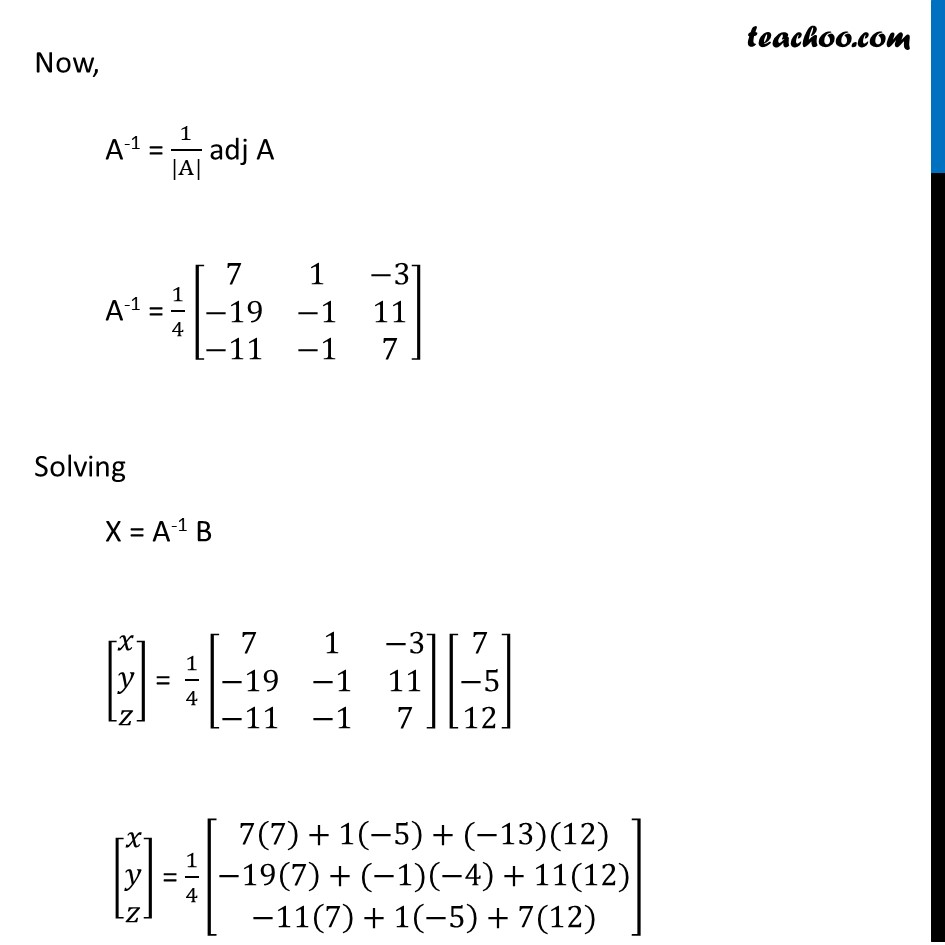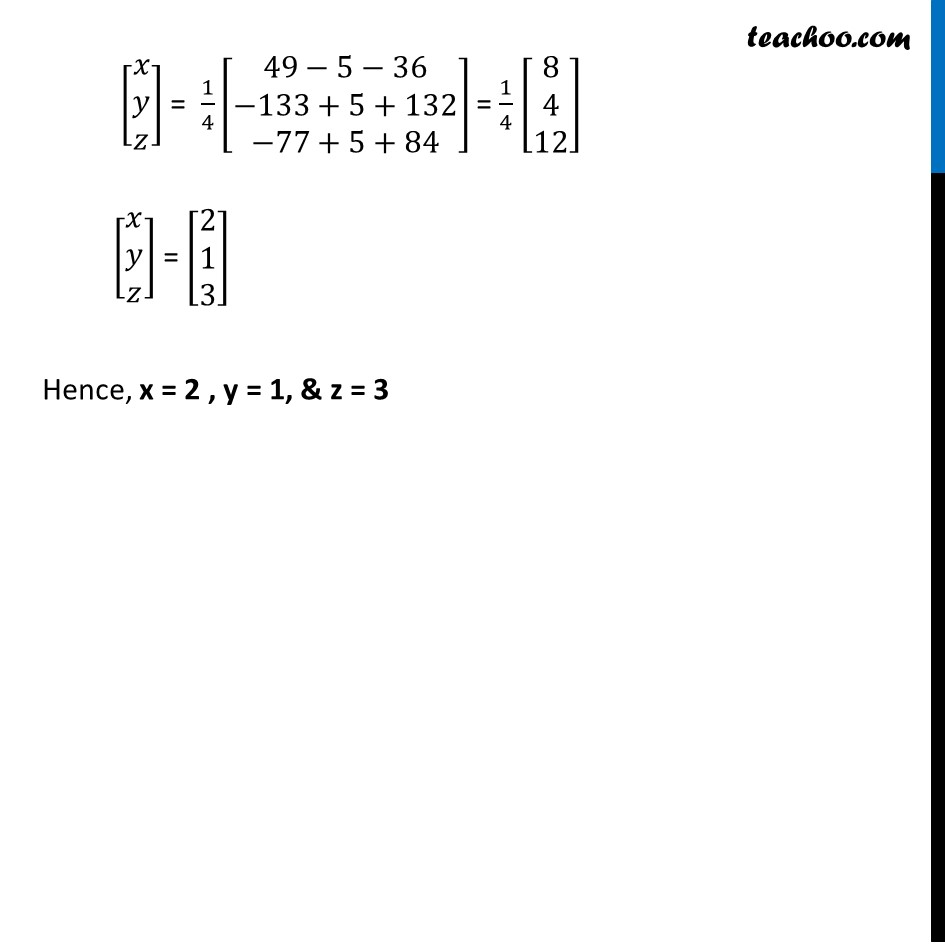Learn in your speed, with individual attention - Teachoo Maths 1-on-1 Class

### Transcript

Ex 4.5, 14 Solve system of linear equations, using matrix method. x − y + 2z = 7 3x + 4y − 5z = −5 2x − y + 3z = 12 The system of equations are x − y + 2z = 7 3x + 4y − 5z = −5 2x − y + 3z = 12 Writing equation as AX = B [■8(1&−1&2@3&4&−5@2&−1&3)] [■8(𝑥@𝑦@𝑧)] = [■8(7@−5@12)] Hence, A = [■8(1&−1&2@3&4&−5@2&−1&3)] , X = [■8(𝑥@𝑦@𝑧)] & B = [■8(7@−5@12)] Calculating |A| |A| = |■8(1&−1&2@3&4&−5@2&−1&3)| = 1 |■8(4&−5@−1&−3)| – ( –1) |■8(3&−5@2&3)| + 2 |■8(3&4@2&−1)| = 1 ( 12 – 5) + 1 (9 + 10) + 2 (–3 – 8) = 2 (7) + 1 (19) + 2 (–11) = 7 + 19 – 22 = 4 Since |A|≠ 0 ∴ The system of equation is consistent & has a unique solution Now, AX = B X = A-1 B Calculating A-1 Now, A-1 = 1/(|A|) adj (A) adj A = [■8(A11&A12&A13@A21&A22&A23@A31&A32&A33)]^′ = [■8(A11&A21&A31@A12&A22&A32@A13&A23&A33)] A = [■8(1&−1&2@3&4&−5@2&−1&3)] M11 = [■8(4&−5@−1&3)] = 12 – 5 = 7 M12 = |■8(3&−5@2&3)| = 9 + 10 = 19 M13 = |■8(3&4@2&−1)| = –3 – 8 = –11 M21 = |■8(−1&2@−1&3)| = −3 + 2 = –1 M22 = |■8(1&2@2&3)| = 3 – 4 = –1 M23 = |■8(1&−1@2&−1)| = –1 + 2 = 1 M31 = |■8(−1&2@4&−5)| = 5 – 8 = –3 M32 = |■8(1&2@3&−5)| = –5 – 6 = –11 M33 = |■8(1&−1@3&4)| = 4 + 3= 7 Now, A11 = 〖"(–1)" 〗^(1+1) M11 = (–1)2 . 7 = 7 A12 = 〖"(–1)" 〗^"1+2" M12 = 〖"(–1)" 〗^"3" . 19 = – 19 A13 = 〖(−1)〗^(1+3) . M13 = 〖(−1)〗^4 . – 11= –11 A21 = 〖(−1)〗^(2+1) M21 = 〖(−1)〗^3 . (–1) = + 1 A22 = 〖(−1)〗^(2+2) M22 = (–1)4 . (–1) = –1 A23 = 〖(−1)〗^(2+3). M23 = 〖(−1)〗^5. 1 = –1 A31 = 〖(−1)〗^(3+1). M31 = 〖(−1)〗^4 . (–3) = –3 A32 = 〖(−1)〗^(3+2) . M32 = 〖(−1)〗^5. (–11) = 11 A33 = 〖(−1)〗^(3+3) . M33 = (–1)6 . (–7) = 7 Thus, adj A = [■8(7&1&−3@−19&−1&11@−11&−1&7)] Now, A-1 = 1/(|A|) adj A A-1 = 1/4 [■8(7&1&−3@−19&−1&11@−11&−1&7)] Solving X = A-1 B [■8(𝑥@𝑦@𝑧)] = 1/4 [■8(7&1&−3@−19&−1&11@−11&−1&7)] [■8(7@−5@12)] [■8(𝑥@𝑦@𝑧)] = 1/4 [■8(7(7)+1(−5)+(−13)(12)@−19(7)+(−1)(−4)+11(12)@−11(7)+1(−5)+7(12))] [■8(𝑥@𝑦@𝑧)] = 1/4 [■8(49−5−36@−133+5+132@−77+5+84)] = 1/4 [■8(8@4@12)] [■8(𝑥@𝑦@𝑧)] = [■8(2@1@3)] Hence, x = 2 , y = 1, & z = 3